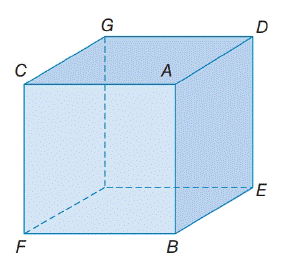Chapter 2.1, Problem 18E### Elementary Geometry for College St...

6th Edition
Daniel C. Alexander + 1 other
ISBN: 9781285195698

#### Solutions

Chapter
Section### Elementary Geometry for College St...

6th Edition
Daniel C. Alexander + 1 other
ISBN: 9781285195698
Textbook Problem
1 views

# In the three-dimensional figure, C A ¯ ⊥ A B ¯ and B E ¯ ⊥ A B ¯ . Are C A ↔   and   B E ↔ parallel to each other? (Compare with Exercise 6.)To determine

To check:

CAandBE parallel to each other or not.

Explanation

Given:

In the three-dimensional figure, CA¯AB¯ and BE¯AB¯.

Figure (1)

Approach:

Parallel lines are lines in the same plane that do not intersect.

Calculation:

The given statement is, CA¯AB¯ and BE¯AB¯

### Still sussing out bartleby?

Check out a sample textbook solution.

See a sample solution

#### The Solution to Your Study Problems

Bartleby provides explanations to thousands of textbook problems written by our experts, many with advanced degrees!

Get Started

#### Simplify: 4854

Elementary Technical Mathematics

#### 21. From the following list of sets, indicate which pairs of sets are disjoint.

Mathematical Applications for the Management, Life, and Social Sciences

#### Solve the equations in Exercises 126. x69x4=0

Finite Mathematics and Applied Calculus (MindTap Course List)